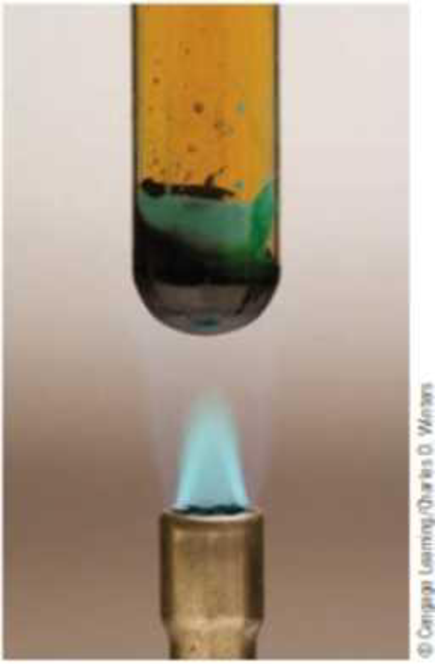Chapter 10, Problem 103IL

Chapter
Section
Textbook Problem

Many nitrate salts can be decomposed by heating. For example, blue, anhydrous copper(II) nitrate produces the gases nitrogen dioxide and oxygen when heated. In the laboratory, you find that a sample of this salt produced a 0.195-g mixture of gaseous NO2 and O2 with a total pressure of 725 mm Hg at 35 °C in a 125-mL flask (and black, solid CuO was left as a residue). What is the average molar mass of the gas mixture? What are the mole fractions of NO2 and O2 in the mixture? What amount of each gas b in the mixture? Do these amounts reflect the relative amounts of NO2 and O2 expected based on the balanced equation? Is it possible that the fact that some NO2 molecules combine to give N2O4 plays a role?Heating copper(II) nitrate produces nitrogen dioxide and oxygen gas and leaves a residue of copper(ll) oxide.

Interpretation Introduction

Interpretation:

Given the statement of reaction molar mass and balanced chemical reaction should be solved and identified.

Concept introduction:

Molar mass: The molar mass of a substance is determined by dividing the given mass of substance by the amount of the substance.

Partial pressure: The mixture of gases each gas has a partial pressure which is the hypothetical pressure of that gas if it alone occupied the entire volume of the original mixture at the same temperature.

Total pressure: The total pressure of a mixture of gases is equal to the sum of the partial pressures of the given component of reaction. In other words this equation can be used to determine the partial pressure of an individual gas in the mixture.

Explanation

Let us consider the Cu(NO3)2 balanced equation has shown below

2Cu(NO3)22CuO+2NO2+12O2

Above the decomposition reaction of 1 mol of Cu(NO3)2 should give the two mole of (NO2) and 12 O2. So the actual mole amount 4.72×103moleofgas

The average molar mass of Cu(NO3)2 has 41.3g/mol

The both product mole fraction

molefractionofχ(NO2)=0.666molefractionofχ(O2)=0

Still sussing out bartleby?

Check out a sample textbook solution.

See a sample solution

The Solution to Your Study Problems

Bartleby provides explanations to thousands of textbook problems written by our experts, many with advanced degrees!

Get Started